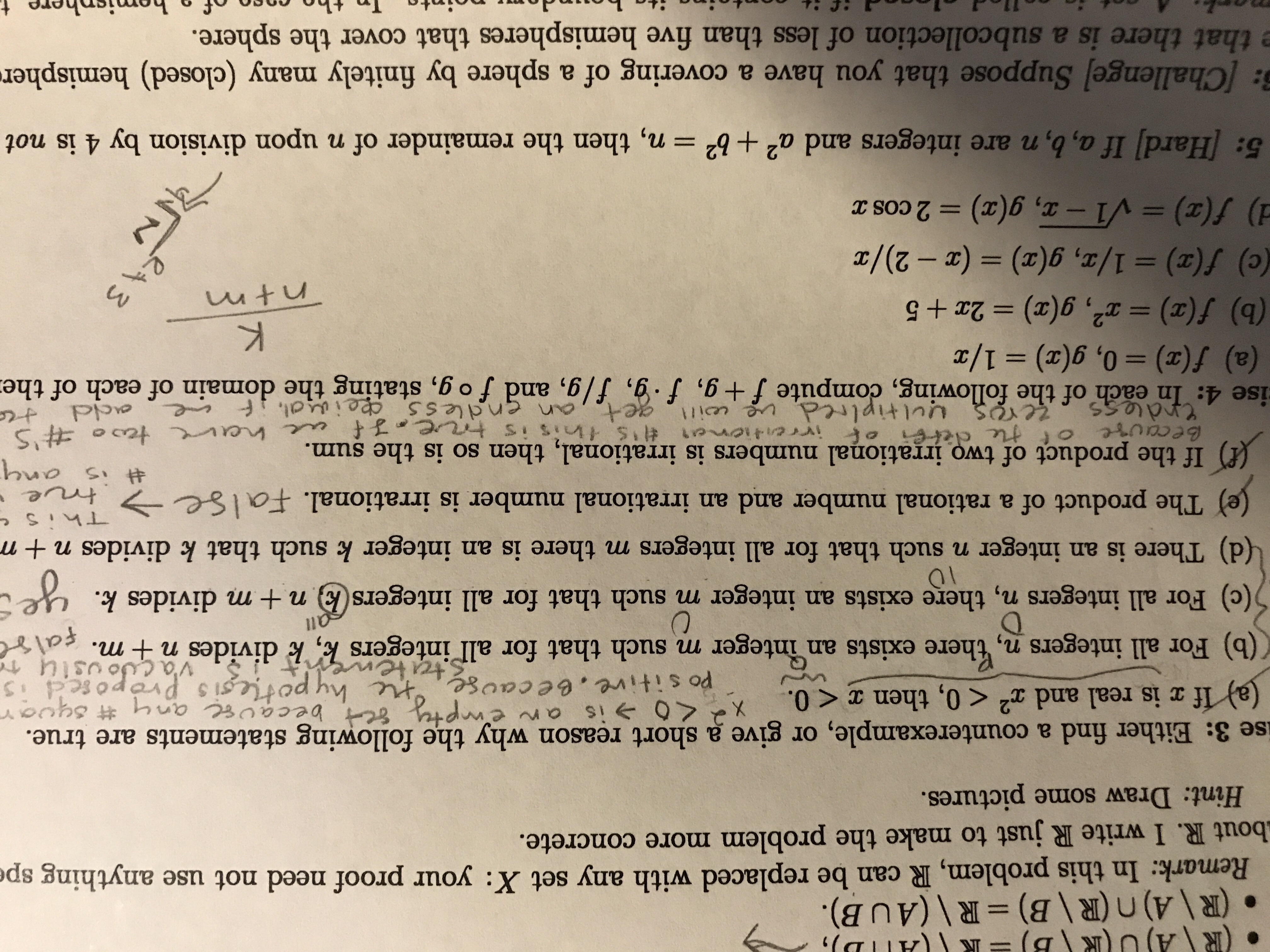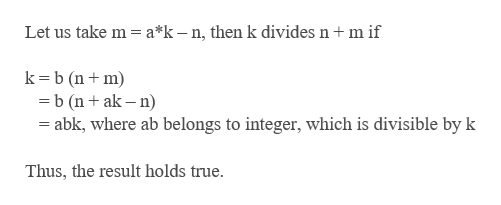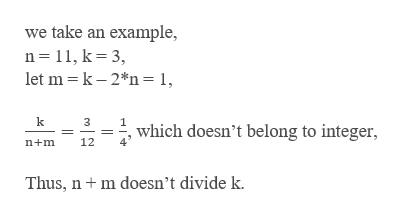# \(R.(R\A)n (R\B) R\ (AU B).Remark: In this problem, R can be replaced with any set X: your proof need not use anything spebout R. I write R just to make the problem more concrete.Hint: Draw some pictures.give a short reason why the following statements are true.X 0 is an enise 3: Either find a counterexample, or(a) If x is real and x2 < 0, then a < 0.Po sitive.ee caus 8 becau any #syvarstatenel Potesis ProrosedJoustu tev'a(b) For all integers n, there exists an integer m such that for all integers k, k divides n + m. falrDU(c) For all integers n, there exists an integer m such that for all integers (k) n + m divides k.10(d) There is an integer n such that for all integers m there is an integer k such that k divides n +mThisCej The product of a rational number and an irrational number is irrational. FalSetrueis anIf the product of two irrentienat 's tnis is trve f e he teo o #St te dete onal numbers is irrational, then so is the sum.becaureCrdless0zeros uultiplred, e oilgetCO,an gndlesS peisal, f eacdise 4: In each of the following, compute f + g, f.g, f/g, and fog, stating the domain of each of the(a) f(x)= 0, g(x) 1/x(b) f(x) r, g(x) 2x +5(e) f(a)= 1/r, g(a) = (z - 2)/) f(z) V1-x, g(a) 2 cos xK11ntmeA 35: Hard] If a, b, n are integers and a2 +b2 = n, then the remainder of n upon division by 4 is not: [Challenge] Suppose that you have a covering of a sphere by finitely many (closed) hemisphere that there is a subcollection of less than five hemispheres that cover the sphere.hemisphere tofTn tho ondoto

Question

Either find a counterexample or give a short reason why:

b) for all integers n, there exists an integer m suck that for all integers k, k divides n+m.

c) for all integers n, there exists an integer m suck that for all integers k, n+m divides k.

d) there is an integer n such that for all integers m there is an integer k suck that k divides n+ m.help_outlineImage Transcriptionclose\ (R . (R\A)n (R\B) R\ (AU B). Remark: In this problem, R can be replaced with any set X: your proof need not use anything spe bout R. I write R just to make the problem more concrete. Hint: Draw some pictures. give a short reason why the following statements are true. X 0 is an en ise 3: Either find a counterexample, or (a) If x is real and x2 < 0, then a < 0. Po sitive.ee caus 8 becau any #syvar statenel Potesis Prorosed Joustu te v'a (b) For all integers n, there exists an integer m such that for all integers k, k divides n + m. falr D U (c) For all integers n, there exists an integer m such that for all integers (k) n + m divides k. 10 (d) There is an integer n such that for all integers m there is an integer k such that k divides n +m This C ej The product of a rational number and an irrational number is irrational. FalSe true is an If the product of two irrentienat 's tnis is trve f e he teo o #S t te dete onal numbers is irrational, then so is the sum. becaure Crdless 0 zeros uultiplred, e oilget CO ,an gndlesS peisal, f e acd ise 4: In each of the following, compute f + g, f.g, f/g, and fog, stating the domain of each of the (a) f(x)= 0, g(x) 1/x (b) f(x) r, g(x) 2x +5 (e) f(a)= 1/r, g(a) = (z - 2)/ ) f(z) V1-x, g(a) 2 cos x K 11 ntm eA 3 5: Hard] If a, b, n are integers and a2 +b2 = n, then the remainder of n upon division by 4 is not : [Challenge] Suppose that you have a covering of a sphere by finitely many (closed) hemispher e that there is a subcollection of less than five hemispheres that cover the sphere. hemisphere t of Tn tho o ndo to fullscreen
check_circleExpert Solution
Step 1

a) For all integer n, there exist an integer m such that for all integers k, k divides n + m.help_outlineImage TranscriptioncloseLet us take m a*k-n, then k divides n m if k b (nm b (nak-n abk, where ab belongs to integer, which is divisible by k Thus, the result holds true. fullscreen
Step 2

b) For all integer n, there exist an integer m suc...help_outlineImage Transcriptionclosewe take an example, n 11, k 3, let m k 2*n = 1 3 which doesn't belong to integer, 12 n+m Thus, n m doesn't divide k. fullscreen

### Want to see the full answer?

See Solution

#### Want to see this answer and more?

Solutions are written by subject experts who are available 24/7. Questions are typically answered within 1 hour*

See Solution
*Response times may vary by subject and question
Tagged in

### Calculus